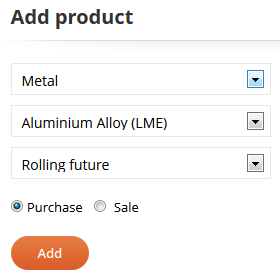﻿ Risk - Kairos Commodities

# Risk

The Risk section is based on Value-at-Risk. VaR operates on several different levels, from the individual source of risk to an entire portfolio of risk. VaR can thus quantify a specific category's exposure or an entire portfolio’s net exposure.

VaR can be used to measure risk across different business areas and products.

VaR is defined as the biggest loss expected for a given confidence interval (e.g. 95%) for a given time period (e.g., a year). That is, there is 5% probability that the loss is bigger than the estimated VaR number in the given period.

The VaR-assesment is done by inputting the estimated consumption of commodities in the given period.

## Create a portfolio

The first step in using Risk is to create your first portfolio. Go to the Risk section and click "Add portfolio".

The portfolio you create will be given a name, based on the date it was created. We recommend that you change the name of your report by clicking on the icon below "Rename".

Click on the icon below "View" to access the portfolio.

## Start the analysisWhen you have opened your portfolio, it will be empty. First add commodities and currencies to the portfolio by clicking on "Add".

This will open a dialogue as shown on the left, where commodities are divided into relevant groups. Choose the commodity or currency you want to add. For some commodities several markets are available, and both futures and spot prices can be included. For others, the options are limited. Finally, choose whether you want this is as a product or currency that you purchase or sell, by clicking on the relevant radio button. Then choose "Add". We recommend you click "Save" to ensure that no data is lost.

## Base variables

You will need to specify the following.

Your company's base currency (default choice is EUR)

The confidence interval (default choice is 95%). You can choose from 80%-99%.

The time horizon in months (default choice is 12 months). You can choose from 1-24 months.

With or without a decay factor (default choice is without). If you choose to calculate the model without the decay factor, then you are choosing a model where the standard deviations are equivalently weighted. Accordingly, when a new price is added to the model, that price deviation from the average will only be weighted the same as the first price deviation in the dataset. This means that the model will adapt to price changes at a slower pace, than if the most recent price deviations were weighted higher. On the other hand, the equivalently weighted model is generally better if the model is calculating data with a long time horizon.

If you choose to calculate the model with the decay factor, then you are choosing a model where the most recent standard deviation is weighted higher than the previous ones. Specifically, by choosing a decay factor of e.g. 94%, the most recent price deviation is weighted 6%, the one before that is weighted 94% of 6%, i.e. 5.64%, the one before that is weighted 94% of 5.64%, i.e. 5.3%, and so on. You may choose a decay factor of 80%-99%.

## Enter data

Once you have selected all base variables and added all commodities and currencies to the portfolio you can enter the relevant consumption or sales data.

For commodities the unit is shown to the right of the input field. E.g. if the unit is "MT", you must specify your consumption in metric tonnes.

After having specified consumption data, remember to click on "Save" to ensure that no data is lost.

## Analysis

In the column "VaR" an individual VaR number. This is the calculated VaR if we were calculating a portfolio that only consisted of the one commodity or currency. But most companies consume more than one commodity. The important VaR calculation is the “Total VaR”, which is the net portfolio risk. This corresponds to the maximum amount in the chosen currency, by which the cost will increase during the chosen time period, at the chosen confidence level.

The column "Comp. VaR", shows a calculation of the component VaR. This is a calculation of the distribution of the net portfolio risk, across the various commodities and currencies included in the calculation.

The column "Marg. VaR" shows a calculation of the marginal VaR. This corresponds to the portfolio effect of spending one unit of currency more on the specific commodity or currency. The calculation is always shown using the base currency, e.g. if the chosen base currency was GBP then the marginal VaR would be calculated in GBP.

Next section: Reports